# SSC Quantitative Aptitude Questions (Day-46)

Dear Aspirants, you can find the Quantitative Aptitude questions with detailed explanations for the SSC exams. Nowadays the competitive level of the exam has been increasing consistently. Due to the great demand for the government job, the level of the toughness reached greater. Candidates have to enhance the preparation process in order to drive in the right path. It doesn’t need to clear the prescribed cutoff. You must have to score good marks more than the cut off marks to get into the final provisional list. Here we have updating the Quantitative Aptitude questions with detailed explanations on a daily basis. You can practice with us and measure your level of preparation. According to that you can sculpt yourself in a proper way. SSC aspirants kindly make use of it and grab your success in your career.

Start Quiz

1) An amount was lent for one year at the rate of 10% per annum compounding annually had the compounding been done half yearly, the interest would have increased by 80. What was the amount (in Rs) lent?

(a) 16000

(b) 32000

(c) 48000

(d) 64000

Direction (2-5): The line chart given below represents the sales (in ’00) of trousers and shirts for five months.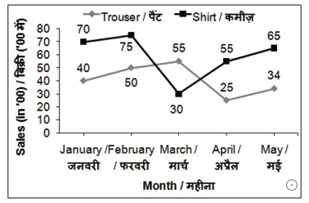2) What is the difference between sales of shirts for months January and April?

(a) 2700

(b) 1500

(c) 2000

(d) 2200

3) What is the percentage increase in the sales of the trousers from January to February?

(a) 25

(b) 20

(c) 10

(d) 28

4) In March sale of trousers is what percent of sale of shirts?

(a) 83.33

(b) 54.54

(c) 125

(d) 183.33

5) Total sale of shirts for five months is how much percent more than the total sale of trousers for five months?

(a) 30.84

(b) 38.46

(c) 44.61

(d) 49.94

6) The ratio of curved surface area of two cones is 1 : 9 and the ratio of slant height of the two cones is 3 : 1. What is the ratio of the radius of the two cones?

(a) 1 : 3

(b) 1 : 9

(c) 1 : 27

(d) 1 : 1

7) If the area of a square is 24, then what is the perimeter of the square?

(a) 2√6

(b) 4√6

(c) 16√6

(d) 8√6

8) What is the total surface area (in cm2) of a cylinder having radius of base as 7 cm and height as 15 cm?

(a) 814

(b) 616

(c) 968

(d) 780

9) A cylindrical well of height 40 metres and radius 7 metres is dug in a field 56 metres long and 11 metres wide. The earth taken out is spread evenly on the field. What is the increase in the level of the field?

(a) 12.5

(b) 10.66

(c) 11.5

(d) 13.33

10) A cuboid of sides 5 cm, 10 cm and 20 cm are melted to form a new cube. What is the ratio between the total surface area of the cuboid and that of the cube?

(a) 6 : 5

(b) 7 : 6

(c) 11 : 10

(d) 9 : 7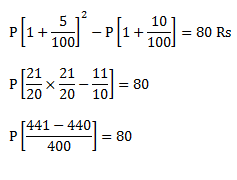P  = 32000

Shirts sold in January = 7000

Shirts sold in April = 5500

Required difference = 1500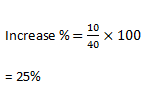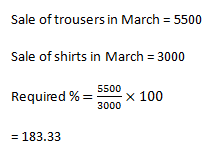Total sale of shirts for five months = 29500

Total sale of trousers for five months = 20400

Required % =  (29500 – 20400)/ 20400 × 100

= 44.61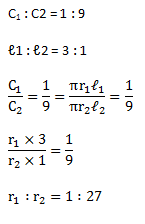a² = 24

a = 2√6

Perimeter = 4a = 4 × 2√6

= 8√6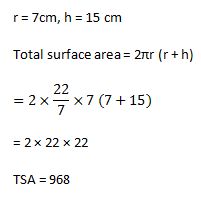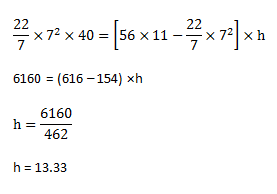Volume of cube = volume of cuboid

a³ = 5 × 10 × 20

a³ = 1000

a = 10 cm

Total surface area of the cuboid = 2(ℓb + bh + hℓ)

= 2 ( 5 × 10 + 10 × 20 + 20 × 5)

= 700 cm²

Total surface area of the cube = 6a²

= 6 × 100

= 600 cm²

Ratio = 7 : 6

 Check Here to View SSC CGL / CHSL 2021 Quantitative Aptitude Questions Day – 45 Day – 44 Day – 43